# CONCAT Function in Excel

Combine text in cells

## What is the CONCAT Function in Excel?

The CONCAT Function in Excel is a text function that is used for combining text in cells. The function was introduced in MS Excel 2016 as a replacement to CONCATENATE function. However, you will find that both functions are available in MS Excel for backward compatibility.

### Formula

=CONCAT (text1,text2….text_n)

### How to use the CONCAT Function in Excel

It is a built-in function that can be used as a worksheet function in Excel. Let’s take an example. You need to combine/concatenate the strings below: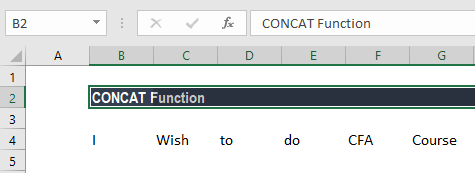So here, we will use the formula below: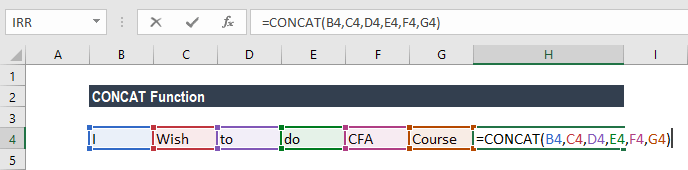Using the formula above, we will get the following result: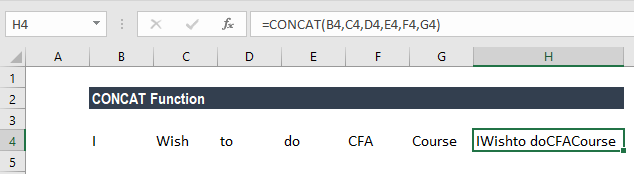You will notice that the above result is not acceptable, as there is no space in between the characters. CONCAT function combines the strings as we instruct it to do. What we got is a long string with the concatenated values running together. Let’s modify this formula to add a space. To do so, we need to add another argument “ ” (two double quotes around a space).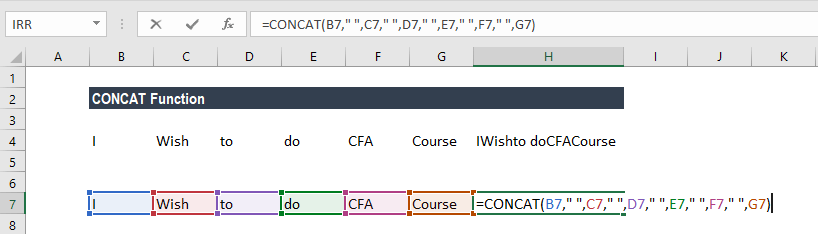Doing so will give us the space required between the characters.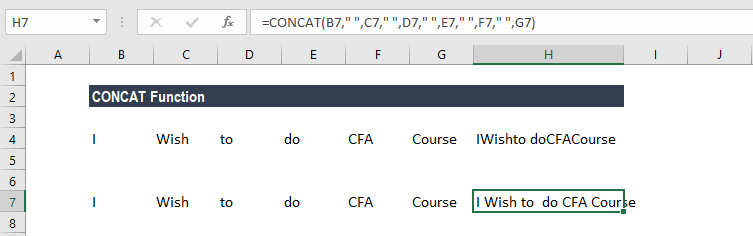In the above formulas, it is necessary to add a comma after each argument or the function will not work.

Let’s consider this example to understand more about how this function works:

Even if the cell contains more than one character, the CONCAT function will combine them as instructed. For example, for the following strings: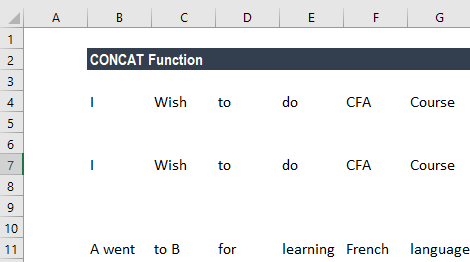When we use the formula CONCAT(B11,” “,C11,” “,D11,” “, E11,” “,F11,” “,G11) it will combine all characters contained in cells B11, C11, D11, E11, F11, and G11 to give us the following result: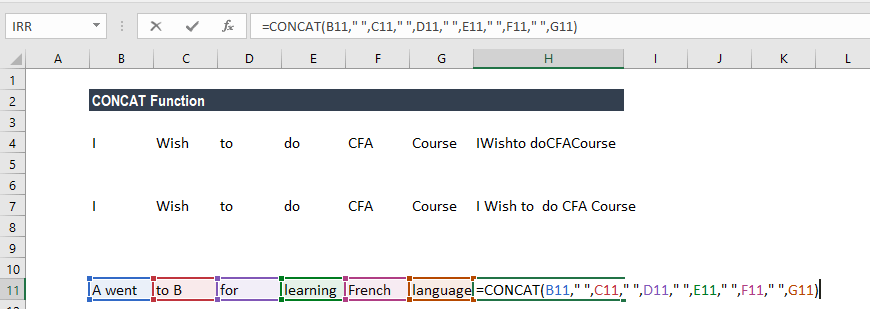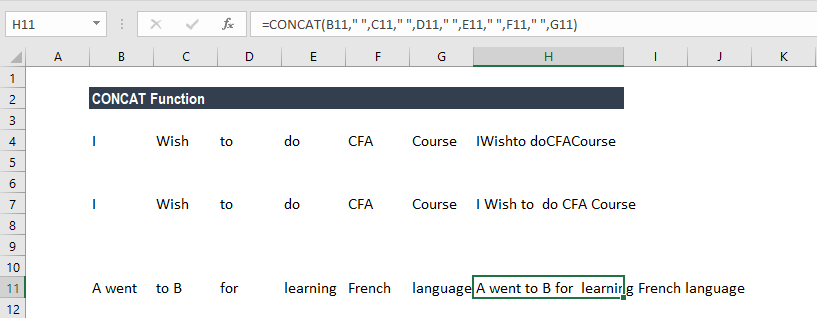### Uses of the CONCAT function

To understand the uses of this function, let’s consider a few examples:

#### Example 1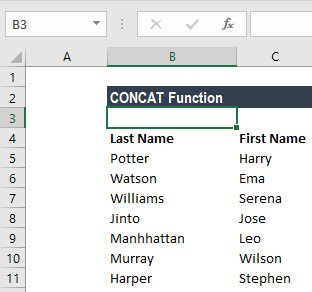However, you need the entire name in one cell and in order of First name, Last name. In this scenario, the CONCAT function can be used.

As we need the first name and then the last name, we would instruct the function to combine the strings in that manner.  As discussed above, the formula to be used would be CONCAT(C5,” “,B5).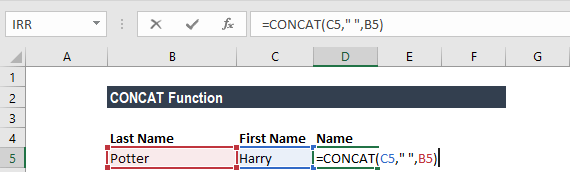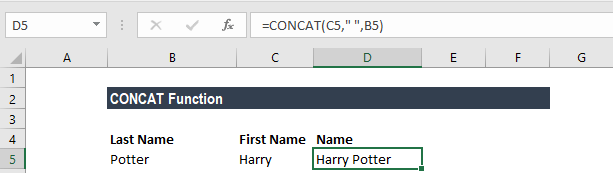After typing the formula in D5, we can drag down the formula for all required cells using fill handle.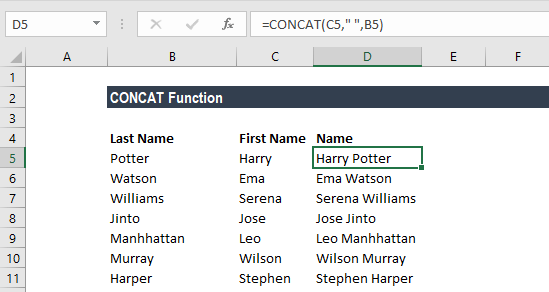#### Example 2

Let’s assume we need the name of suppliers but in capital letters. CONCAT can be used along with other Excel functions to get the desired results. Now in our example, we need the name Harry Potter to be combined but in upper case. In such a scenario, we would use the formula: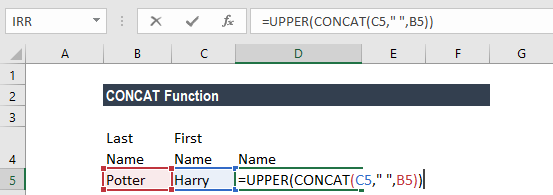The result will be the name in all capital letters: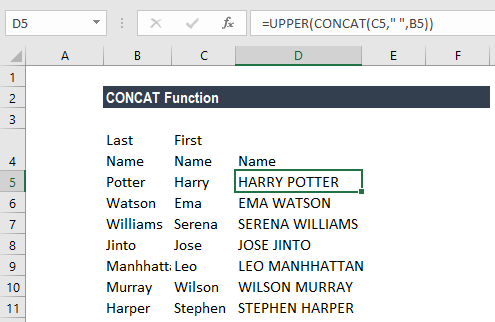#### Example 3

Let’s assume in this example that the suppliers gave you the quotation per meter of the cloth you need as follows: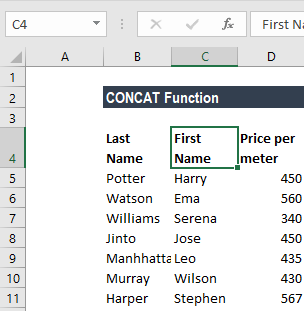Now, you need the data as follows:

Harry Potter, \$450/meter

In such a scenario, the formula to use will be: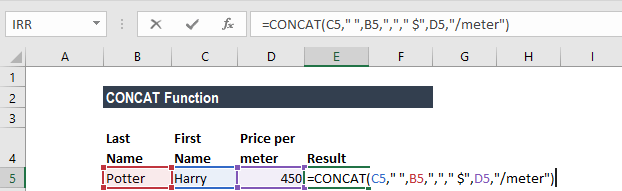So here we used CONCAT formula to combine strings and create a sentence with the additional information.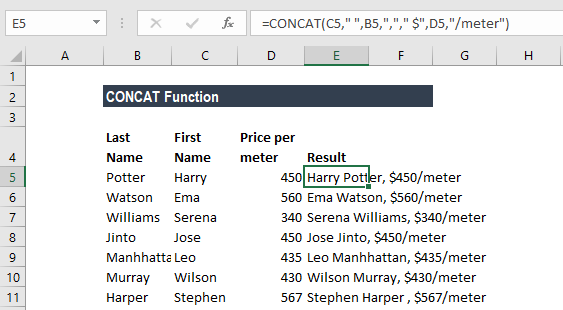#### Example 4

Suppose we wish to add the name, email ID, and address with line breaks, we need to use the CONCAT function along with the CHAR function. Let’s see how:

The formula to use will be =C5 & ” ” & B5 & CHAR(10) & C2 & CHAR(10) & E5 & “, ” & F5 & ” ” & G5

In the above formula, we created a line break through CHAR 10 and merged values were separated using a comma (“, “) and space (” “).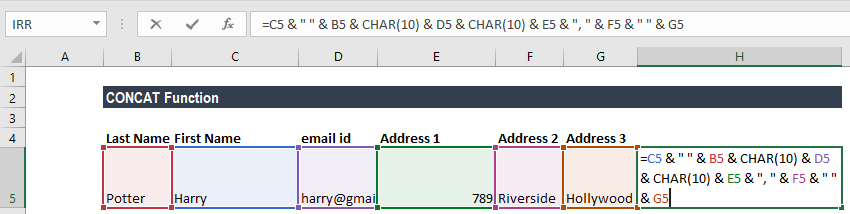The result will be: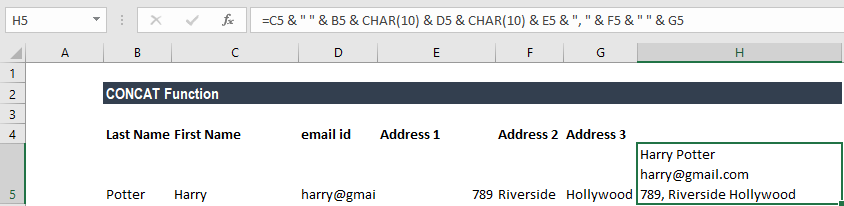We can use wrap text to ensure the results are displayed correctly as shown below: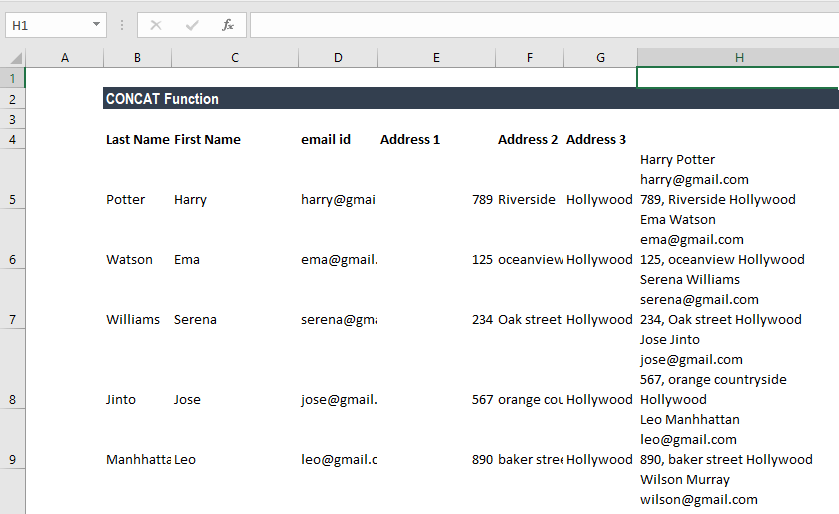### Common Errors in the CONCAT function

As we saw above, if we do not use spaces in between the text to separate text entries, the text entries will run together. So, while using the CONCAT function, we need to add that extra space. To add the space, we add double quotation marks with a space between them ” “.

1. Sometimes quotation marks will appear in the result. This happens when we forget to add a comma. For example, when we input formula =CONCAT(“Hello “”August”) we would get the result as Hello”August with an extra quote mark. The reason as mentioned is that we didn’t add space between the arguments.
2. Numbers can be used with or without quotation marks
3. Reasons for #NAME? error. This error usually occurs when we have missed the quotation mark in the text argument.
4. Reasons for #VALUE! Error. This error will occur when the resulting string exceeds 32,767 characters (cell limit).
5. This function doesn’t recognize arrays so we need to list the cell references separately.
6. Apart from double quotes, forward slash (/) and asterisk (*) can be used to separate the concatenated strings with other characters.

Thanks for reading CFI’s guide to important Excel functions! By taking the time to learn and master these functions, you’ll significantly speed up your financial analysis. To learn more, check out these additional CFI resources:

• Excel Functions for Finance